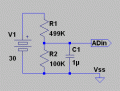# Is the microcontroller's ADC input resistance in parallel or series with the pin?

Joined Dec 15, 2015
1
I am currently working with stm32f407 microcontroller board. I need to sample the ac voltage and for that i need a voltage divider. For that voltage divider to work properly, i need to consider the input resistance of adc. I have found the value of sampling switch resistance to be 6k in datasheet but i am not able to understand whether it is in series or parallel with the input pin of microcontroller? plz help

#### Papabravo

Joined Feb 24, 2006
20,385
I would not put a voltage divider on an A2D input pin. The reason is that this value, whatever it may be, is not tightly specified. The better solution is to implement the divider and couple it to the A2D input with a unity gain follower.

#### MikeML

Joined Oct 2, 2009
5,444
I have done this on PICs and Arduinos with no problems. The input "impedance" of the AD input pin is ~ 1uA of DC current leakage to the Vss pin. The AD input usually specifies that it be driven from a source impedance that is no higher than 10KΩ while the AD is actually converting. You can satisfy this constraint by making the Thev. Equiv of the voltage divider less than 10KΩ.

To save power in battery-powered equipment, I have made the voltage divider Thev. eq. as high as ~80KΩ, but I shunted the AD input pin with a low-leakage 1uF capacitor. This satisfies the low AC source-impedance requirement, while making an anti-alias filter at the same time...

Like this:#### ErnieM

Joined Apr 24, 2011
8,362
As I read that spec (ADC characteristics) the RAIN (4) External input impedance is a maximum value you can present and still achieve good fidelity with your input.

If I found the correct spec their number is 50K. You can safely use a divider as long as the value of the two resistors in parallel (which is the Thevinin equivalent of the divider) is 50K or less.

As long as the series sum does not load what you measure too greatly then you can go with the divider.

Only if you need a higher impedance would you need an op amp buffer. Buffers cost more than good stable resistors and have problems of their own.